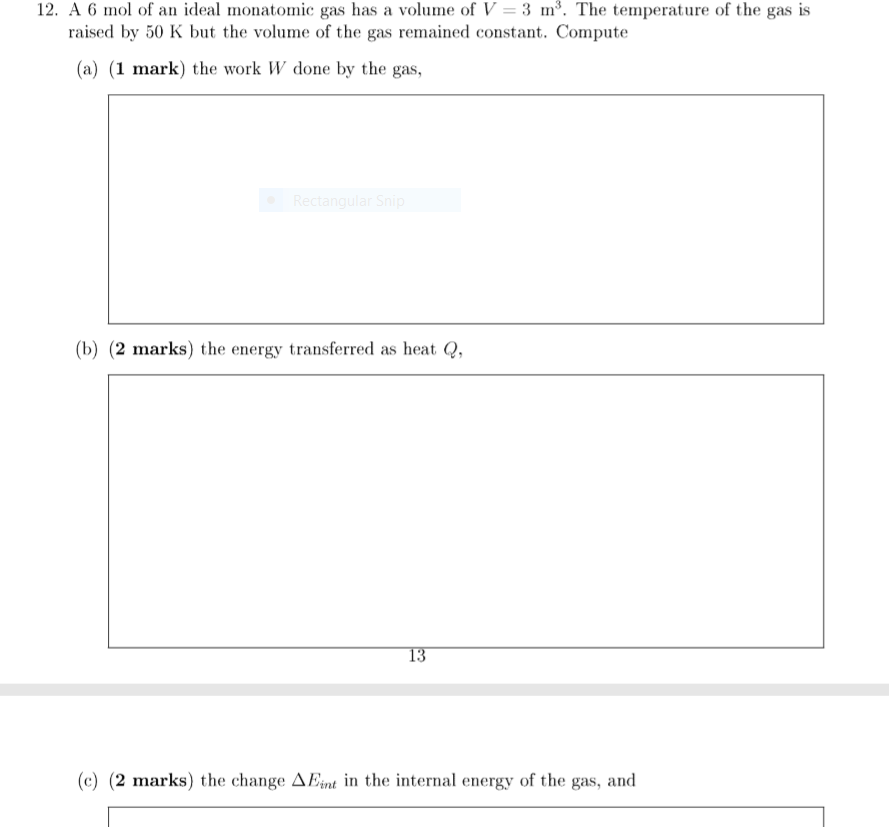# Question Solved1 Answer12. A 6 mol of an ideal monatomic gas has a volume of V = 3 my. The temperature of the gas is raised by 50 K but the volume of the gas remained constant. Compute (a) (1 mark) the work W done by the gas, (b) (2 marks) the energy transferred as heat Q, (c) (2 marks) the change AEint in the internal energy of the gas, andTranscribed Image Text: 12. A 6 mol of an ideal monatomic gas has a volume of V = 3 my. The temperature of the gas is raised by 50 K but the volume of the gas remained constant. Compute (a) (1 mark) the work W done by the gas, (b) (2 marks) the energy transferred as heat Q, (c) (2 marks) the change AEint in the internal energy of the gas, and
More
Transcribed Image Text: 12. A 6 mol of an ideal monatomic gas has a volume of V = 3 my. The temperature of the gas is raised by 50 K but the volume of the gas remained constant. Compute (a) (1 mark) the work W done by the gas, (b) (2 marks) the energy transferred as heat Q, (c) (2 marks) the change AEint in the internal energy of the gas, and